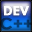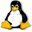Home Latest News Archive Tutorials C++ 2 3 4 Win32 Java OpenGL GLUT OpenGL ES 2 3 4 DirectX VB.NET HTML Photoshop 3dsmax Forums Projects Modelling Links About Us Contact Search Recent Tutorials C / C++ Win32 OpenGL OpenGL ES GLUT## Typecasting

Tutorials > C++ > Typecasting

### Introduction

This tutorial will deal with typecasting, data type suffixes and the representation of certain types of data.

### What is typecasting?

Generally you will be dealing with a number of different data types. If you try to assign a value of one data type to another data type, this may cause issues. The compiler will try to do this automatically but it may produce errors or warnings. An example of an automatic typecast occurs when you place a variable within a true / false statement. If your variable is equal to 0, then it is false, otherwise it is true. To force the compiler to convert this data, a typecast is used. This is achieved by placing the name of the required data type in brackets before the data that you are wanting to convert.

A typecast is usually not necessary when casting from a variable of smaller size to a variable of greater size eg. from a short to an int. A typecast is necessary to convert a float to an int as an int can obviously not show any decimal places.

Contents of main.cpp :

```#include <iostream>
#include <stdlib.h>

using namespace std;

int main()
{
```

We define a number of variables below to use for our typecasting. Notice that an f is placed after the value given to the float. The compiler automatically sees a decimal value as a double. Placing an f on the end specifies that the value is a float value. This prevents compiler warnings.

```	char c = 'A';
int i = 45450;
long l = 122;
float f = 45.45f;
double d = 323.23;
```

Our first typecast involves casting an int to a short. A short can not hold a value of 45450. A value of -20086 is therefore produced due to overflow. An overflow occurs when you move past the maximum value of a data type. The value basically wraps around to the minimum value of that data type.

```	cout << (short)i << endl;
```

Our next typecast displays the ascii code for an A. This value is 65.

```	cout << (int)c << endl;
```

If we convert a number to a char eg. from long to char, we get the ascii character associated with that number. In this case, the ascii character for 122 is z.

```	cout << (char)l << endl;
```

Our next typecast deals with decimal values. If you convert from a decimal number to one without decimals eg. from float to int, the decimal part is basically truncated from the number. This is an easy way to round down from a current decimal value.

```	cout << (int)f << endl;
```

As was seen above, you can place a suffix on the end of a number to specify what type the number represents. As was seen with the f, an l can be placed after a number to represent a long value.

```	l = 4000l;
f = 300.0f;
```

Although this does not relate directly to typecasting, it may be useful to your programs. If you wish to output a hexidecimal number, you can use the format 0xyyyy where yyyy is a 4 digit number eg. 0x3233 yields a value of 12851. If you want to specify an exponent the same way as a calculator (power of 10), you can use the e character within the number eg. 1.2e5 represents 1.2 times 10 to the power of 5 which is 120000.

```	cout << 0x3233 << endl;
cout << 1.2e5 << endl;

system("pause");

return 0;
}```

Congratulations. You should now be able to convert the basic data types to each other.

Please let me know of any comments you may have : Contact Me

 Source Files :< Tutorial 28 - Advanced Structures Tutorial 30 - Command Line Arguments >

All Rights Reserved, © Zeus Communication, Multimedia & Development 2004-2005

Read the Disclaimer